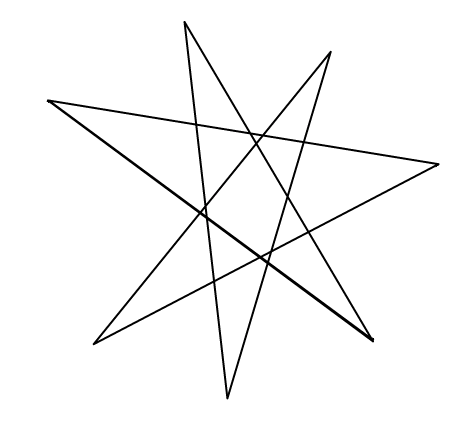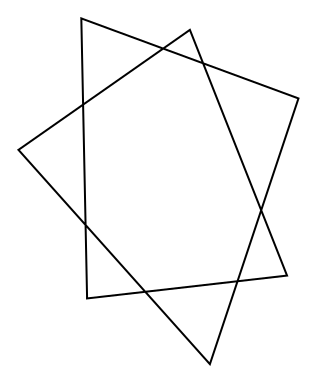#### You may also like### Getting an Angle

How can you make an angle of 60 degrees by folding a sheet of paper twice?### Arclets Explained

This article gives an wonderful insight into students working on the Arclets problem that first appeared in the Sept 2002 edition of the NRICH website.### Bow Tie

Show how this pentagonal tile can be used to tile the plane and describe the transformations which map this pentagon to its images in the tiling.

# Star Polygons

### Why do this problem?

This problem offers a nice opportunity for students to practise measuring angles in a situation that leads them to make a discovery that they can then try to prove.

### Possible approach

Show the image of the five-pointed star from the problem or draw one, and invite students to draw stars of their own. Ask them to measure the angles at the points as accurately as they can, and then add the five angles together. Collect some of their results on the board.
"It looks like the answers are all around $180^{\circ}$. I wonder if we can prove it?"

There are a number of options for proving the result. Students could create a diagram in GeoGebra and use it to gain insights about how the different angles change when they change the star.
Students who are fluent in algebra could be encouraged to label the angles in their diagram and use angle rules to write down relationships between the angles.
Some students may wish to consider the special case of a "regular" star whose points lie at five equally spaced positions on a circle.

Bring the class together to discuss proof methods and share ideas. Then perhaps go onto the seven-pointed star. The animation in the problem shows one way of proving the result for a seven-pointed star. The total of the angles in the 7 triangles is the same as the sum of the interior angles of the heptagon and twice the sum of the angles at the points of the star.

Alternatively, some students may wish to consider the angle turned through as they mentally "walk" around the lines of the star.

### Key questions

If you know the five interior angles of the pentagon, what other angles can you work out?
What do you know about the angle sum of a pentagon?
Could algebra help?

### Possible support

Polygon Pictures offers students practice in calculating angles in regular polygons.

### Possible extension

Students could investigate stars with more points, or different types of stars made by joining points around a circle differently. For example, the two stars below have been created by plotting seven points around a circle, and in the first image joining every third point, and in the second image joining every second point.